NumberSpiral.com

CONTENTS8.  Factor curves

It turns out that every integer on a product curve is intersected by a second related curve.

The existence of the second curve is a consequence of the following general property: the product of any two adjacent integers on a product curve can be found further out on that curve at a distance from the first factor which is equal to the first factor's value.

Here's an example:

 4   5   8   13   20   29   40   53...

We can regard the first pair of integers, 4 and 5, as the factors "4 x 5." To find their product, count "0, 1, 2, 3, 4" starting with the 4. You land on 20.

Try again with the second pair, "5 x 8." Count from 0 to 5 starting with the 5, and you land on 40.

This works with every pair of integers on every product curve on the spiral.

As a consequence of this, every integer on a product curve is intersected by a second curve that has the factors explicitly present on it. The intersection of the two curves is like a visual statement of the multiplication: the product lies on one curve and the factors lie on the other. When a curve plays the second role, I call it a "factor curve." (Only a small fraction of curves are product curves, but every curve is a factor curve with respect to some of the integers on it.)

The relationship between the two curves at the intersection is interesting. Let's look at an example:VortexTo draw these angles with Vortex, enter "fac 4 6" in the command window.

Figure 1

 The product in this example is 24. The blue product curve is S–1, indicating a difference of 2, so the factors are 4 x 6. Those factors appear in order on the red factor curve. Counting up from 4 on the red curve (starting with zero), we arrive on the count of 4 at 24 on the blue curve. It all works out neatly. But that's not all. There is a relationship between the adjacent integers on one curve and the adjacent integers on the other. On one curve those numbers are 15 and 35; on the other, 16 and 34. The difference between corresponding numbers is one. The intersection of the two curves seems to contain a great deal of information in geometric form about the factorization. I'll close this page with several more illustrations of intersections (two each from S, P, P–2, and S–4):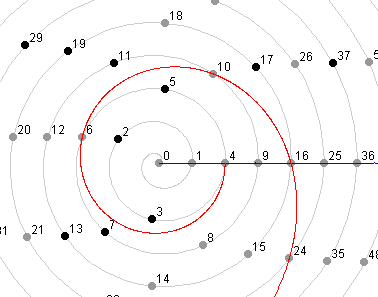VortexTo draw these angles with Vortex, enter "fac 4 4" in the command window.

Figure 2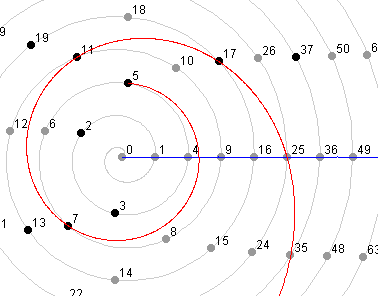VortexTo draw these angles with Vortex, enter "fac 5 5" in the command window.

Figure 3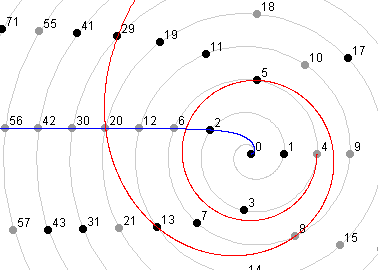VortexTo draw these angles with Vortex, enter "fac 4 5" in the command window.

Figure 4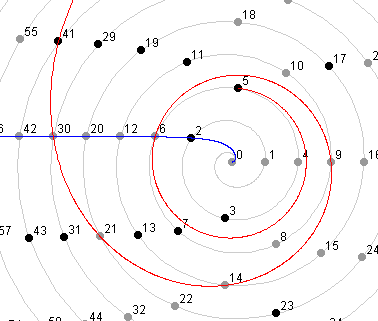VortexTo draw these angles with Vortex, enter "fac 5 6" in the command window.

Figure 5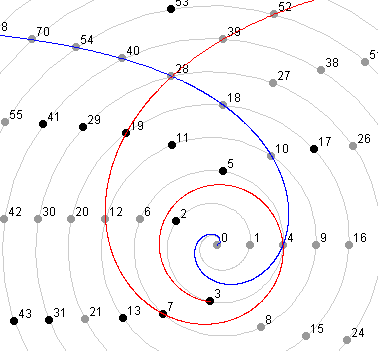VortexTo draw these angles with Vortex, enter "fac 4 7" in the command window.

Figure 6VortexTo draw these angles with Vortex, enter "fac 5 8" in the command window.

Figure 7VortexTo draw these angles with Vortex, enter "fac 4 8" in the command window.

Figure 8VortexTo draw these angles with Vortex, enter "fac 5 9" in the command window.

Figure 9Back  1  2  3  4  5  6  7  8  9  10  Next